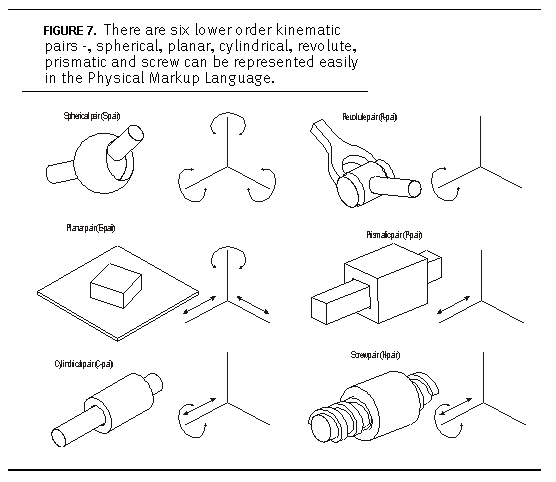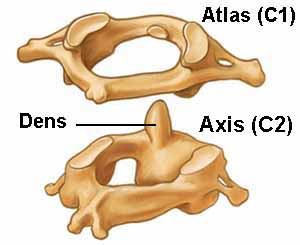Morphology
W.S.Harwin

# Degrees of freedom

• Serial chains
• e.g. most extremities (arms/legs)
• Parallel chains
• e.g. rib cage
• Steward platform
• mixed
• e.g. delta robot

## Grueblers equation and Kutzbach criterion

Relates degrees of freedom to the links and joints.

### Planar

$m=3(n-j-1)+\sum^j f_i$

### Spatial

$m=6(n-j-1)+\sum^j f_i$

where $n$=number of links, $j$= number of joints, $f_i$ = d.o.f. in joint $i$, $m$=degrees of freedom Usually need to have at least one actuator per degree of freedom

# The lower pairs

Six lower order kinematic pairs

 name DoF type Revolute 1 R Prismatic 1 P Screw 1 R+P Cylindrical 2 RT spherical/Ball 3 RRR Planar 3 RRTIn fact the screw is the most general robot joint. All rigid motion of links in a robot can be described as a combination of screw motions.

Question, how does a screw joint represent

• a revolute joint
• a prismatic joint?

# Anatomical joints# Robot reachable workspace

Robot (and human) workspace is limited by

• Joint range of movement
• boundary singularities (can't move beyond the workspace boundary)
• internal singuularities (joint axes come into alignment)

Singularities are where the Jacobian is singular, (in this case the Jacobian is a location dependent relationship beween the joint veolcity and the endpoint velocity) $\Delta x=J \Delta\theta$

So if J is singular, then to achieve a specific $\Delta x$ requires an infinite joint velocity vector.

Likewise $\tau=J^T F$ where $\tau$ is the joint torque and $F$ is the end point force so at a workspace singularity there is no ability of the joints to opose the external force

# Affordance and morphology

• concept of affordance in robotics is championed by Rolf Pfeifer (Zurich University), that the morphology is exploited by the central nervous system

Examples

• Foldable robots (see papers for review)
• Flexible (snake/octopus/salamander robots) e.g. Auke Jan Ijspeert EPFL
• Climbing/Jumping/Cockroach/Gecko robots (e.g. Robert J Full Berkeley)
• morphology for specific applications, any number of robot grippers are designed for the task (eg industrial assembly and packing, agriculture). Compare these with the capabilities of the human hand.